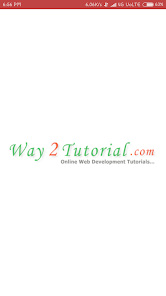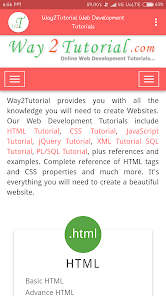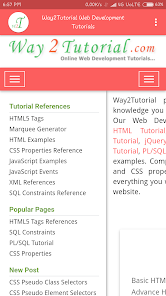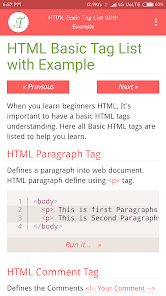# HTML, CSS, JS, jQuery, XML, SQL, PLSQL Tutorials

10K+EveryoneWay2tutorial - The Prime App to Learn Interactive Web Development Tutorials, examples, and references completely Free

HTML Tutorial
CSS Tutorial
JavaScript Tutorial
jQuery Tutorial
AJAX Tutorial
XML Tutorial
SQL Tutorial
PL/SQL Tutorial

HTML Tutorial Features
2. This HTML tutorial will teach you the latest HTML5 standards.
3. Learn HTML with 40+ HTML Lessons.
4. Every lesson are detailed explanations that help you effectively learn HTML.
5. Learn HTML with 150+ HTML examples.
6. Try It Yourself with every example.
7. List out all HTML tags references.

CSS Tutorial Features
1. Learn CSS3 tutorials, CSS properties.
2. All CSS lesson are updated as latest standards of CSS.
3. Learn CSS with 45+ CSS Lessons.
4. Every lesson are step by step explain that help you quickly learn CSS.
5. Learn CSS with 120+ CSS examples.
6. Try It Yourself with every CSS example.
7. List out all CSS properties reference.

JavaScript Tutorial Features
1. Completely free learn JavaScript tutorials.
2. All JavaScript lesson are updated as per latest ECMAScript 6 standard.
3. Learn JavaScript with 40+ JavaScript Lesson.
4. Every lesson are explain step by step that help you to understand quickly.
5. Learn JavaScript with 75+ JavaScipt examples.
6. Try It Yourself with every JavaScript example.

jQuery Tutorial Features
3. Learn jQuery with 40+ jQuery Lessons.
4. Every jQuery lesson are explain with demo example that will help you more effectively.
5. Learn jQuery with 75+ jQuery examples.
6. Try It Yourself with every jQuery example.

Ajax Tutorial Features
1. Learn Ajax with 10+ Ajax Lessons with examples.
2. Try It Yourself with every jQuery example.

XML, DTD, XPATH Tutorial Features
1. Learn XML, DTD, XPATh tutorials on this App.
2. Learn XML with 10+ XML Lessons.
3. Learn DTD with 10+ DTD Lessons.
4. Learn XPATH with 10+ XPATH Lessons.
5. Every XPATH lesson are explain step by step with example.

SQL Tutorial Features
2. Learn SQL with 45+ SQL Lessons with examples.
3. Every SQL tutorial lesson explain step by step with sample SQL Query examples.
4. Quick learn SQL Constraints, SQL Joins, SQL functions.

PL/SQL Tutorial Features
2. Learn PL/SQL with 15+ SQL Lessons with examples.
3. Every PL/SQL lesson explain step by step with sample example.
4. You will learn PL/SQL data types, conditions, looping, type of cursor, how to handling PL/SQL exceptions, how to define PL/SQL functions, procedures, packages, and PL/SQL Trigger.